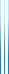# PublicationsManuscripts
Yaroslav Bulatov asked on mathoverflow what is the asymptotic number of self-avoiding integer walks of length $n$ in which adjacent positions are either 1 or 2 apart. We obtain a formula for the exact number of such walks, and deduce that it is $\tilde{O}(\mu^n)$, where $\mu \approx 2.20556943040059$.